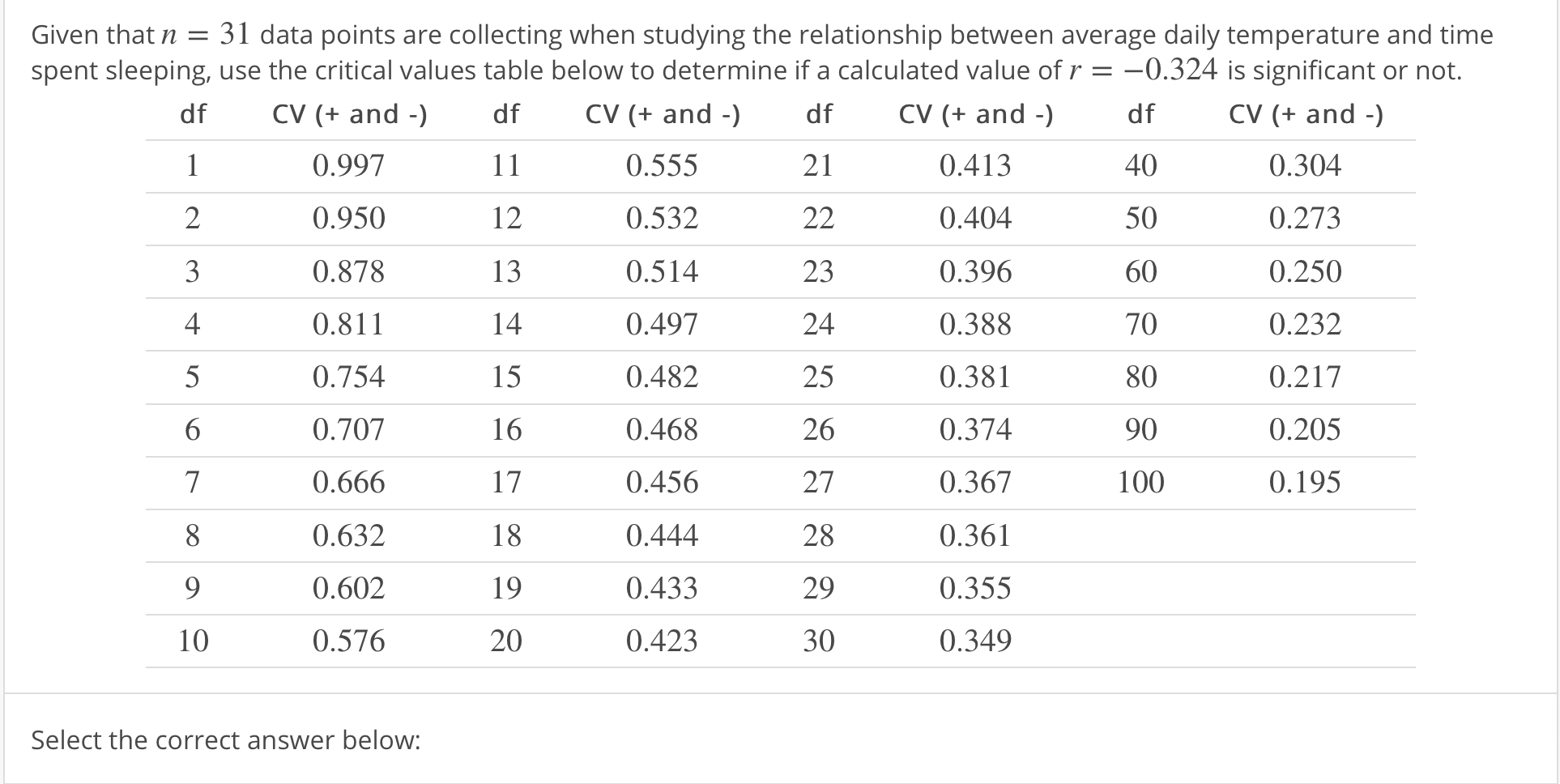# Given that n = 31 data points are collecting when studying the relationship between average daily temperature and timespent sleeping, use the critical values table below to determine if a calculated value of r =-0.324 is significant or not.CV (+ and -)0.3040.2730.2500.2320.2170.2050.195df405060708090100df CV (+ and0.9970.9500.8780.8110.7540.7070.6660.6320.6020.576df CV (+ and - dfCV (+ and -)0.5550.5320.5140.4970.4820.4680.4560.4440.4330.4230.4130.4040.3960.3880.3810.3740.3670.3610.3550.3491223413232425262728293015161718192010Select the correct answer below:

Question
1 views

r is significant because it is between the positive and negative critical values.

r is not significant because it is between the positive and negative critical values.

r is significant because it is not between the positive and negative critical values.

r is not significant because it is not between the positive and negative critical values.help_outlineImage TranscriptioncloseGiven that n = 31 data points are collecting when studying the relationship between average daily temperature and time spent sleeping, use the critical values table below to determine if a calculated value of r =-0.324 is significant or not. CV (+ and -) 0.304 0.273 0.250 0.232 0.217 0.205 0.195 df 40 50 60 70 80 90 100 df CV (+ and 0.997 0.950 0.878 0.811 0.754 0.707 0.666 0.632 0.602 0.576 df CV (+ and - dfCV (+ and -) 0.555 0.532 0.514 0.497 0.482 0.468 0.456 0.444 0.433 0.423 0.413 0.404 0.396 0.388 0.381 0.374 0.367 0.361 0.355 0.349 12 2 3 4 13 23 24 25 26 27 28 29 30 15 16 17 18 19 20 10 Select the correct answer below: fullscreen
check_circle

Step 1

State the appropriate hypothesis:

The hypotheses are given below:

Null hypothesis:

H0 : ρ = 0

That is, there is no significant relationship between the variables.

Alternative hypothesis:

H1 : ρ ≠ 0 (Two tail test).

That is, there is a significant relationship between the variables.

Step 2

Given information:

The sample size is n = 31,

The sample correlation coefficient is r = –0.324.

The degrees of freedom is d.f = n –2 = 31–2 = 29.

Step 3

Obtain the critical value:

The critical value is obtained from the given table, by using the following procedure:

• Locate the value 29 in the column, named f.
• Move towards the right along the row of 29, and find the value corresponding to the column, named CV(+ and &n...

### Want to see the full answer?

See Solution

#### Want to see this answer and more?

Solutions are written by subject experts who are available 24/7. Questions are typically answered within 1 hour.*

See Solution
*Response times may vary by subject and question.
Tagged in

### Other